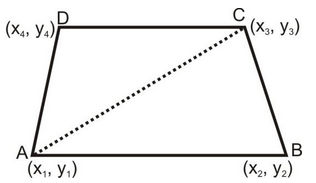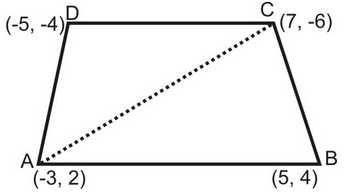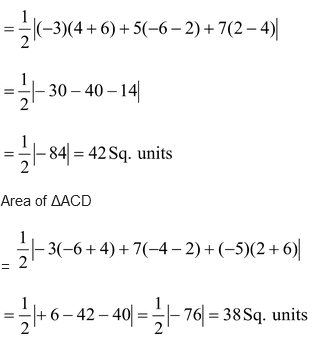## Formula for Area of Quadrilateral

Area of quadrilateral = (½) × diagonal length × sum of the length of the perpendiculars drawn from the remaining two vertices.

Let the vertices of quadrilateral ABCD are A(x1, y1), B(x2,y2), C(x3,y3) and D(x4, y4)

So, area of the quadrilateral ABCD = area of Δ ABC + area of Δ ACDQ 1. Find the area of quadrilateral whose vertices, taken in order, are A(-3, 2), B(5, 4), C(7, -6) and D(–5, –4).

Ans: Area of the quadrilateral ABCD = area of ΔABC + area of ΔACDSo, area of ΔABCSo, area of the quadrilateral ABCD = 42 + 38 = 80 Sq. units.